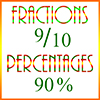# Basics of Fractions / PercentagesIn mathematics, fractions and percentages are both rational numbers. These numbers are called “rational”, not because they’re more reasonable than other numbers, but because they are formed as the ratio of two integers. For example, 2/5, 25/8, -4/3, and 0 are all rational numbers. Every integer is a rational number, because it can be expressed as a ratio with 1 as its denominator. Percentages are also rational, because every percentage is merely a fraction with 100 as its denominator. Rational numbers are also real numbers, but since there are also irrational numbers, not all real numbers are rational.

Ratios are sometimes written in the form m:n, which is equivalent to the fraction m/n. Improper fractions are fractions whose numerator is larger than its denominator, like 14/3. Mixed numbers are numbers which are a combination of an integer and a proper fraction, like 4 2/3.

## Equivalent Fractions

A fraction consists of an integer numerator divided by a non-zero integer denominator. Two fractions are equivalent if they reduce to the same fraction when expressed in lowest terms. This can be done as follow:

Consider the two fractions 12/28 and 15/35. Starting with 12/28, take the prime factorization of both its numerator and its denominator.

12/28 = 2²·3/2²·7

You can see that both numerator and denominator have 2² as a factor, and so these cancel out, leaving

3/7

Now do the same with 15/35.

15/35 = 3·5/5·7

In this case, both numerator and denominator have 5 as a factor. Cancelling these out, we get

3/7

And so 12/28 = 15/37 = 3/7.

A proportion is a statement of equality between two fractions or ratios. Thus, a proportion problem might be x/2 = 8/4, for which x = 4.

Two fractions can be added or subtracted only if they have the same denominator. If two fractions have different denominators we can transform them into equivalent fractions with the same denominator, and then we can add or subtract them. For example, consider 5/6 and 4/15. Taking the prime factorization of both denominators, we get:

6 = 2·3
15 = 5·3

Now we multiply 5/6 by 1 in the form 5/5:

5/6 × 5/5 = 25/30

and we multiply 4/15 by 1 in the form 2/2:

4/15 × 2/2 = 8/30

Now we can add and subtract 5/6 and 4/15 by using equivalent fractions:

5/6 + 4/15 =
25/30 + 8/30 = 33/30 = 11/10

5/6 – 4/15 =
25/30 – 8/30 = 17/30

## Multiplication and Division

Multiplication of fractions is very simple. You just multiply numerators and denominators – that is, the numerator of the product of two fractions is the product of their numerators, and the denominator is the product of their denominators. Example: 5/6 and 4/15.

5/6 × 4/15 = 5×4/6×15 = 20/90 = 2/9

Division is slightly harder and may seem like a mathematical trick. If you divide 5/6 by 4/15, you first turn 4/15 upside down, and then you multiply:

5/6 ÷ 4/15 =
5/6 × 15/4 = 75/24 = 25/8

Why turn the divisor upside down? This is done because when a fraction is turned upside down, it becomes the inverse or the reciprocal of the original fraction:

4/15 × 15/4 = 1

and division is the same as multiplying by the inverse.

## Percentages

Percentages are simply shorthand for writing fractions whose denominators are 100. Thus, 75% = 75/100.

Notice that since the denominator in every percentage is 100, then every percentage can be written as a decimal, so:

75% = 75/100 = .75
38% = 38/100 = .38
215% = 215/100 = 2.15
2% = 2/100 = .02
.2% = .2/100 = 2/1000 = .002

This last example fudged a little, because the numerator is a decimal instead of an integer. But the principle is the same – we’re dividing .2 by 100, which is the same as dividing 2 by 1000. Also, be careful with percentages that have decimals within them, as in this example. We might be inclined to think .2% is the same as 2% or as 20%, but it’s not. It’s 2/10 of 1%, which is much smaller than 2% or 20%.

### Addition, Subtraction, Multiplication, and Division of Percentages

Since percentages can be expressed as decimals, then the arithmetic operations performed on them are the same as they are for decimals. See decimal arithmetic.

Sample Fraction and Percentage Problems